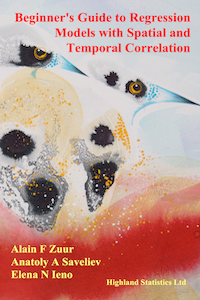www.highstat.com
Highland Statistics Ltd

Latest news

Starting in June, July and August 2020: Online courses with on-demand video and live Zoom meetings:

• Data Exploration, Regression, GLM & GAM with an introduction to R.
• Linear Mixed-Effects Models and GLMM with R-INLA.
• Introduction to Regression Models with Spatial Correlation using R-INLA.
• Introduction to Zero Inflated Models using R-INLA.
• Zero-inflated GAMs and GAMMs for the analysis of spatial and spatial-temporal correlated data using R-INLA.

See highstat.com for details.### Beginner's Guide to Spatial, Temporal and Spatial-Temporal Ecological Data Analysis with R-INLA (2017).  Zuur, Ieno, Saveliev

This book consists of two volumes.

In Volume I we explain how to apply linear regression models, generalised linear models (GLM), and generalised linear mixed-effects models (GLMM) to spatial, temporal, and spatial-temporal data. The models that will be employed use the Gaussian and gamma distributions for continuous data, the Poisson and negative binomial distributions for count data, the Bernoulli distribution for absence–presence data, and the binomial distribution for proportional data.

In Volume II we apply zero-inflated models and generalised additive (mixed-effects) models to spatial and spatial-temporal data. Volume II is entitled  GAM and Zero-Inflated Models.